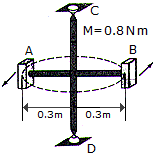# Engineering Mechanics - KOP: Impulse and Momentum - Discussion

### Discussion :: KOP: Impulse and Momentum - General Questions (Q.No.19)

19.The two blocks A and B each have a mass of 500 g. The blocks are fixed to the horizontal rods and their initial velocity is 2 m/s in the direction shown. If a couple moment of M = 0.8 N • m is applied about CD of the frame, determine the speed of the block in 4 s. The mass of the supporting frame is negligible and its free to rotate about CD.

 [A]. v2 = 10.67 m/s [B]. v2 = 23.3 m/s [C]. v2 = 22.3 m/s [D]. v2 = 12.67 m/s

Explanation:

No answer description available for this question.